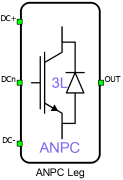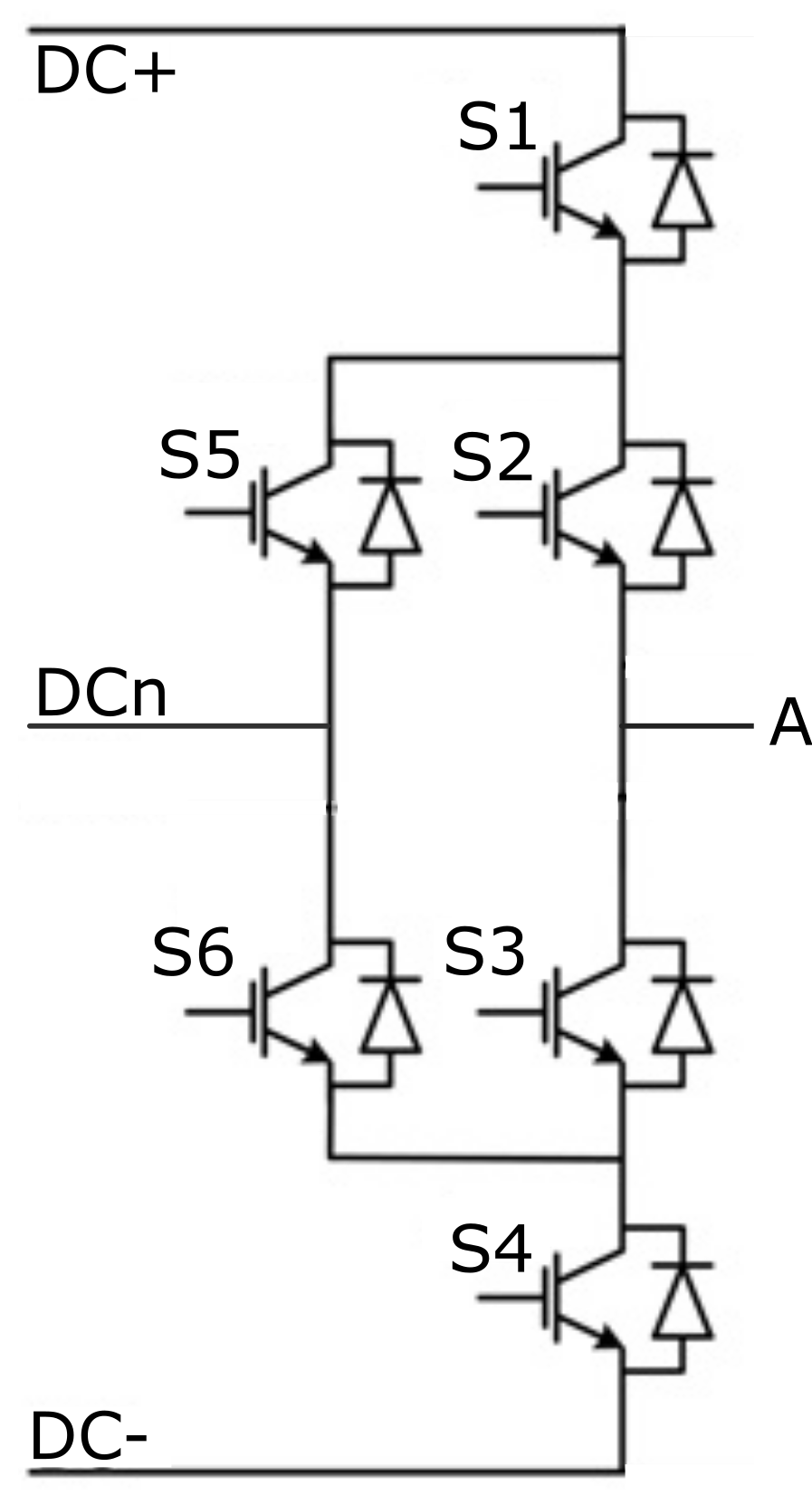# ANPC Leg

Description of the ANPC Leg component in Schematic Editor

A block diagram and input parameters for an ANPC Leg block are given in Table 1.

Table 1. ANPC Leg block in the Schematic Editor core library
component component dialog window component parametersANPC Leg• General
• Control(Digital inputs, Model)
• S1 (1..32(64))
• S2 (1..32(64))
• S3 (1..32(64))
• S4 (1..32(64))
• S5 (1..32(64))
• S6 (1..32(64))
• gate_logic (active high, active low)
• Gate control enabling (enables usage of external digital signal to enable the switching)
• Sen (digital input selection for PWM enable)
Weight = 1

A schematic block diagram of the inverter switching block is given in Figure 1 with corresponding switch arrangement and naming.

Digital inputs, when selected as the Control parameter, enables you to assign gate drive inputs to any of the digital input pins (from 1 to 32(64)). For example, if S1 is assigned to 1, the digital input pin 1 will be routed to the S1 switch gate drive. In addition, the gate_logic parameter selects either active high (High-level input voltage VIH turns on the switch), or active low (Low-level input voltage VIL turns on the switch). The gate drive logic depends on your external controller design.

Model, when selected as the Control parameter, enables you to set the IGBTs' gate drive signals directly from the signal processing model. The input pin gates appears on the component. It requires a vector input of six gate drive signals in the following order: [S1, S2, S3, S4, S5, S6]. When controlled from the model, the logic is always set to active high.

Gate control enabling, when checked, enables use of an external PWM enabling digital signal.Figure 1. A schematic block diagram of an ANPC Leg block diagram with corresponding switch naming

## PESB Optimization

The PESB Optimization option is available in certain converter models. When PESB Optimization is enabled, all converter's short circuit state space modes will be merged and treated as the same state space mode. For example, if one converter leg within the three phase converter is short circuited and PESB Optimization is enabled, all of the legs within the three phase converter will also be short circuited. This simplification for short circuit modeling can save a significant amount of matrix memory.

## Losses calculation

When the Losses calculation property is enabled, the component will calculate switching and conduction power losses for all switching elements (IGBTs and Diodes or MOSFETs). In the case of MOSFET switching elements, the diode characteristic represents the internal MOSFET body diode. Switching power losses are calculated as a function of current, voltage, and temperature using 3D lookup tables. Also, 2D input for losses is supported. When a 2D losses table is inserted, it assumes only current (I) and temperature dependence. Conduction power losses can be defined as a function of current and temperature using Vt and Vd lookup tables (LUTs). These LUTs can be 1D or 2D tables. If the LUT is a 1D table, forward voltage drop depends only on the current, but if LUT is a 2D table, forward voltage drop dependence on the junction temperature is also considered. In the MOSFET case under reverse current conduction, a current sharing calculation between the MOSFET channel and the internal body diode is performed. Import options and an explanation how to correctly fill all necessary power losses parameters is described in the Importing power losses data section. All switches are distributed in three groups, and for each group, different power loss parameters can be specified (S1 and S4 are in group 1, S2 and S3 are in group 2, S5 and S6 are in group 3).

In the case of the IGBT switch type in all groups, input/output terminals for power losses receive/generate vectors of 12 elements (indexes 0-11, where every index in the vector represent one switching element). The ordering of switching elements is described in Table 2.

Table 2. Switching elements ordering for power losses terminals in the case of IGBT switch type in all groups
Index in the vector Switching element
 S1_igbt
 S1_diode
 S2_igbt
 S2_diode
 S3_igbt
 S3_diode
 S4_igbt
 S4_diode
 S5_igbt
 S5_diode
 S6_igbt
 S6_diode

In the case of the MOSFET switch type in all groups, input/output terminals for power losses receive/generate vectors of 6 elements (indexes 0-5, where every index in the vector represent one switching element). The ordering of switching elements is described in Table 3:

Table 3. Switching elements ordering for power losses terminals in the case of MOSFET switch type in all groups
Index in the vector Switching element
 S1_mosfet
 S2_mosfet
 S3_mosfet
 S4_mosfet
 S5_mosfet
 S6_mosfet

If the MOSFET switch type is used for losses group 1 and IGBT switch type for losses groups 2 and 3, input/output terminals for power losses are vectors of ten elements (every index in the vector represent one switching element). Ordering of switching elements is given in Table 4.

Table 4. Switching elements ordering for power losses terminals in the case of MOSFET switch type in group 1 and IGBT switch type in groups 2 and 3
Index in the vector Switching element
 S1_mosfet
 S2_igbt
 S2_diode
 S3_igbt
 S3_diode
 S4_mosfet
 S5_igbt
 S5_diode
 S6_igbt
 S6_diode

If the MOSFET switch type is used for losses groups 1 and 2 and the IGBT switch type for losses group 3, input/output terminals for power losses are vectors of eight elements (every index in the vector represents one switching element). Ordering of switching elements is given in Table 5.

Table 5. Switching elements ordering for power losses terminals in the case of MOSFET switch type in groups 1 and 2 and IGBT switch type in group 3
Index in the vector Switching element
 S1_mosfet
 S2_mosfet
 S3_mosfet
 S4_mosfet
 S5_igbt
 S5_diode
 S6_igbt
 S6_diode

If the MOSFET switch type is used for losses groups 1 and 3 and IGBT switch type for losses group 2, input/output terminals for power losses are vectors of eight elements (every index in the vector represent one switching element). Ordering of switching elements is given in Table 6.

Table 6. Switching elements ordering for power losses terminals in the case of MOSFET switch type in groups 1 and 3 and IGBT switch type in group 2
Index in the vector Switching element
 S1_mosfet
 S2_igbt
 S2_diode
 S3_igbt
 S3_diode
 S4_mosfet
 S5_mosfet
 S6_mosfet

If the MOSFET switch type is used for losses group 2 and IGBT switch type for losses groups 1 and 3, input/output terminals for power losses are vectors of ten elements (every index in the vector represent one switching element). Ordering of switching elements is given in Table 7.

Table 7. Switching elements ordering for power losses terminals in the case of MOSFET switch type in group 2 and IGBT switch type in groups 1 and 3
Index in the vector Switching element
 S1_igbt
 S1_diode
 S2_mosfet
 S3_mosfet
 S4_igbt
 S4_diode
 S5_igbt
 S5_diode
 S6_igbt
 S6_diode

If the MOSFET switch type is used for losses groups 2 and 3 and IGBT switch type for losses group 1, input/output terminals for power losses are vectors of eight elements (every index in the vector represent one switching element). Ordering of switching elements is given in Table 8.

Table 8. Switching elements ordering for power losses terminals in the case of MOSFET switch type in groups 2 and 3 and IGBT switch type in group 1
Index in the vector Switching element
 S1_igbt
 S1_diode
 S2_mosfet
 S3_mosfet
 S4_igbt
 S4_diode
 S5_mosfet
 S6_mosfet

If the MOSFET switch type is used for losses group 3 and IGBT switch type for losses groups 1 and 2, input/output terminals for power losses are vectors of ten elements (every index in the vector represent one switching element). Ordering of switching elements is given in Table 9.

Table 9. Switching elements ordering for power losses terminals in the case of MOSFET switch type in group 3 and IGBT switch type in groups 1 and 2
Index in the vector Switching element
 S1_igbt
 S1_diode
 S2_igbt
 S2_diode
 S3_igbt
 S3_diode
 S4_igbt
 S4_diode
 S5_mosfet
 S6_mosfet

Available component properties are:

• Losses groups - Switching elements group

• Current values - Switching elements current axis [A]
• Voltage values - Switching elements voltage axis [V]

• Temp values - Switching elements temperature axis [°C]

• Vt table - Switch forward voltage drop, f(I,T) [V]

• Vd table - Diode forward voltage drop, f(I,T) [V]

• Et on table - Switch switching ON losses, output energy, f(I, V, T) [J]

• Et off table - Switch switching OFF losses, output energy, f(I, V, T) [J]

• Ed off table - Diode switching OFF losses, output energy, f(I, V, T) [J]

## Temperatures calculation

When the Temperatures calculation property is enabled, the component will calculate the combined power losses (P_loss) and junction temperatures (T_junctions) for all switching elements (IGBTs and diodes). Combined power losses represent the sum of the calculated switching and conduction losses transfered through the internally generated Thermal network component. The internally generated Thermal network component also calculates junction temperatures from power losses, input case temperatures and provided thermal model parameters. For each switching group different thermal parameters can be specified. Input/output ports for temperature calculation are vectors whose number of elements depends on the selected switch types. They are indexed in the same way as explained in Losses calculation.

Additional temperature calculation component properties are:

• Thermal networks type - Defines type of internal thermal network
• Rth switch - List of thermal resistance for the IGBT switch
• Tth switch / Cth switch - List of thermal time constants or thermal capacitances for the IGBT switch
• Rth diode - List of thermal resistances for the diode
• Tth diode / Cth diode - List of thermal time constants or thermal capacitances for the diode
• Calculation execution rate - Execution rate in [s] for the losses and temperature calculation logic

## Digital Alias

If a converter is controlled by digital inputs, an alias for every digital input used by the converter will be created. Digital input aliases will be available under the Digital inputs list alongside existing Digital input signals. The alias will be shown as Converter_name.Switch_name, where Converter_name is name of the converter component and Switch_name is name of the controllable switch in the converter.